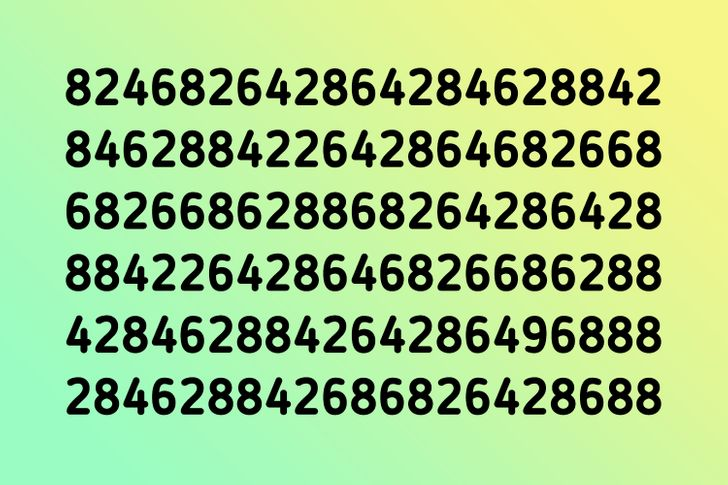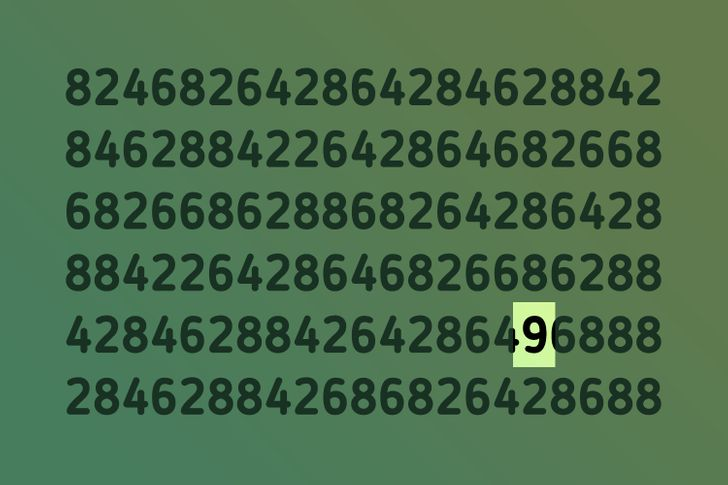# Test: Find the Odd One Out in Less Than 10 Minutes

Puzzles not only give you some leisure time but also help you develop better skills in solving daily issues. In fact, science says that the skills we actually use to work on daily problems are the same we use to find the odd one out in an image.

We at Bright Side have a new collection of puzzles that, with good focus, you might be able to solve in no more than 10 minutes.

### 1. Find the odd cat.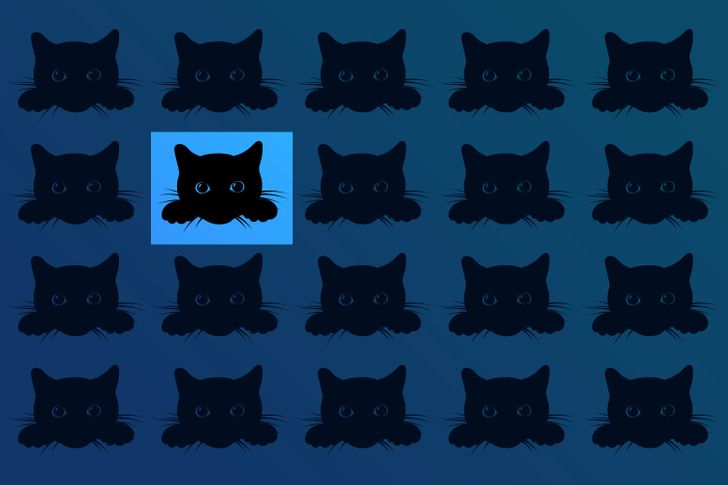### 2. Find the odd fork.### 3. Where is the different paw?### 4. Which one stands out?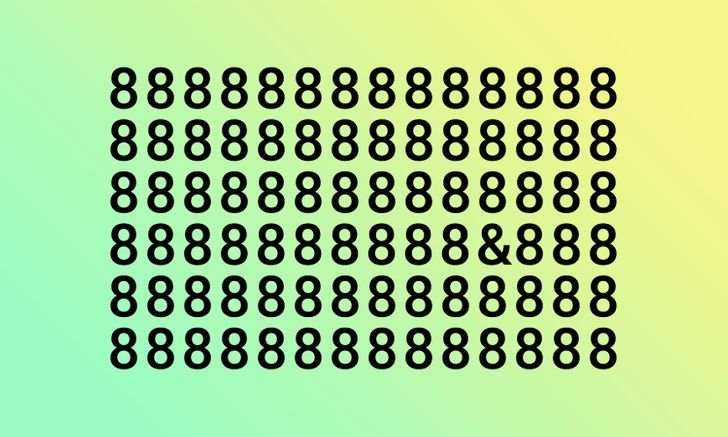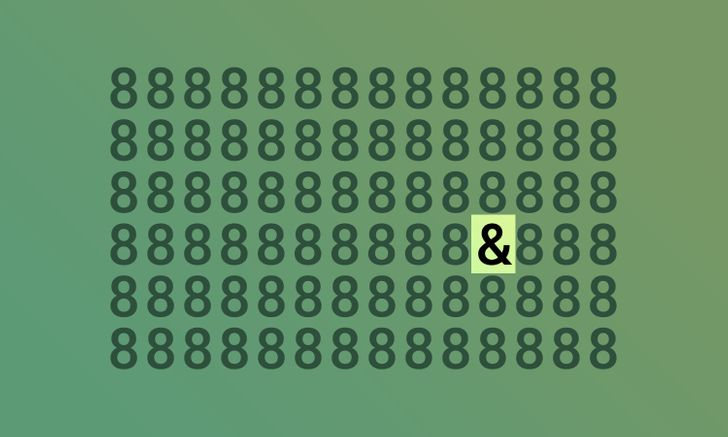### 5. Find the one that doesn’t belong here.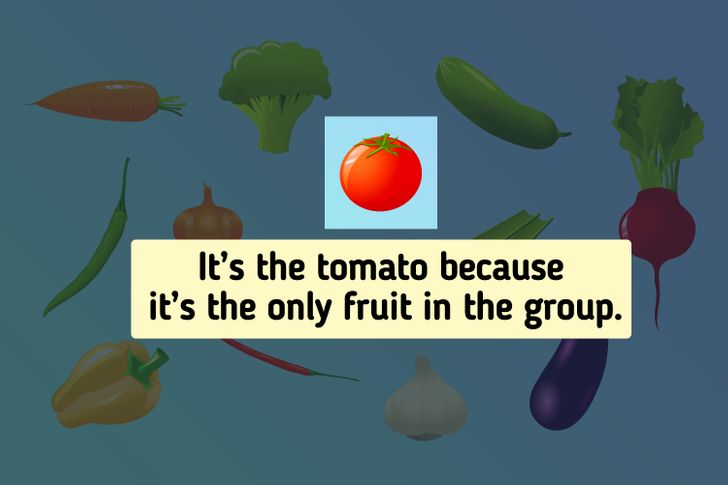### 6. Find the odd fruit.### 7. Find the “Y”.### 8. Find the odd flower.### 9. Find the odd lion.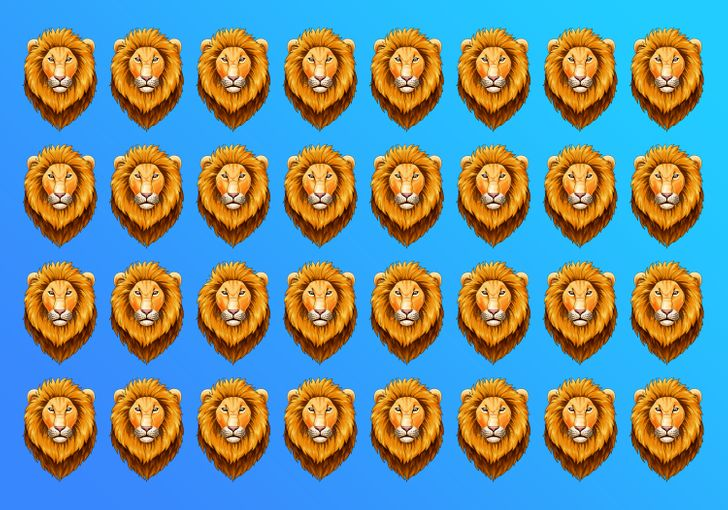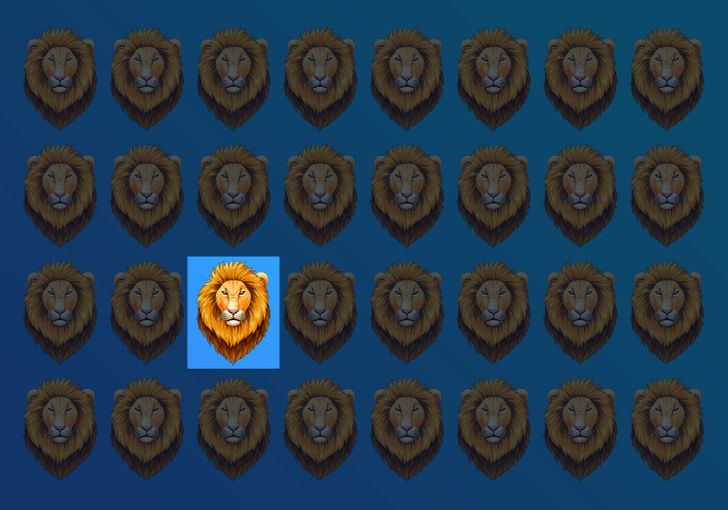### 10. Which one doesn’t belong on the list?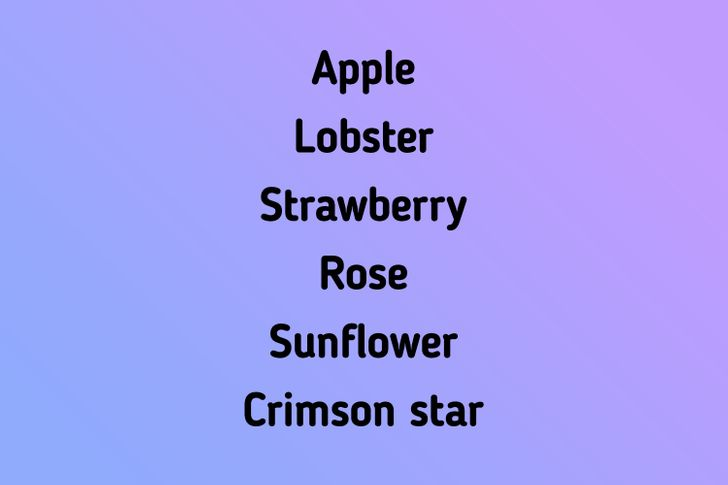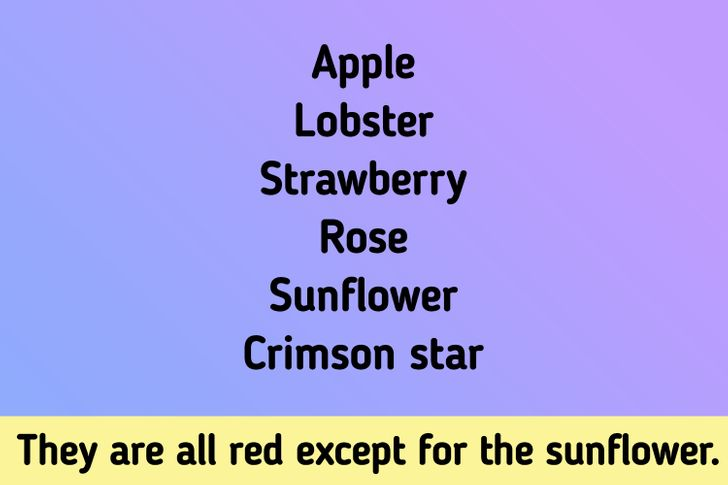### 11. Find the animal that doesn’t belong here.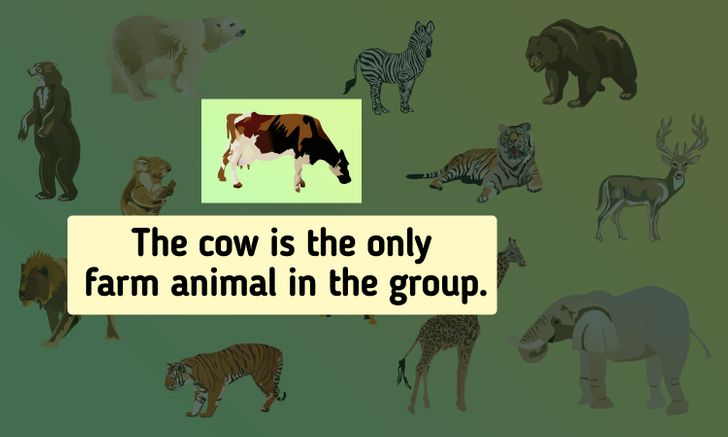### 12. Find the sunflower.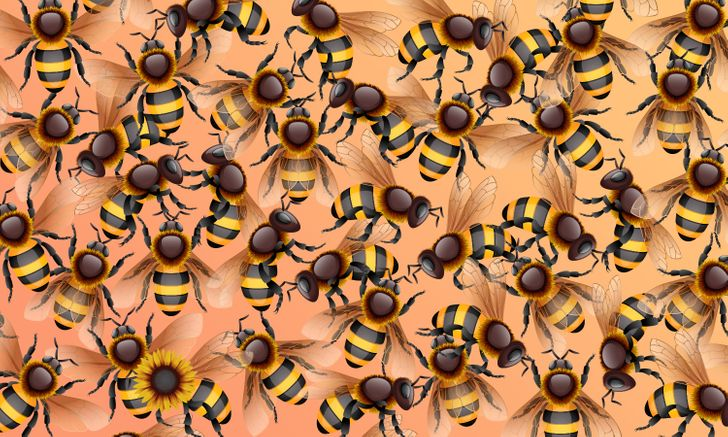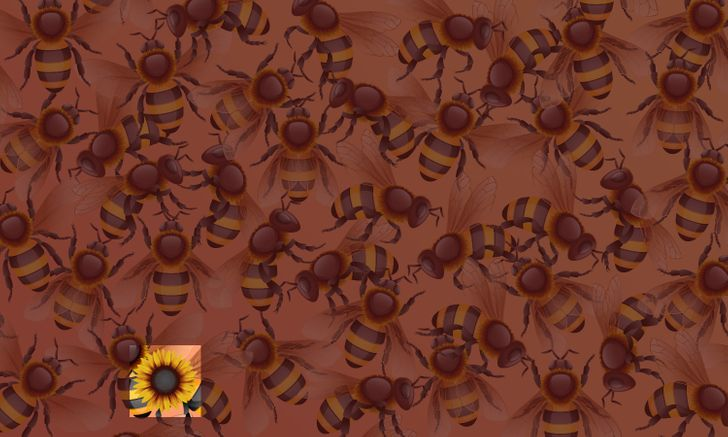### 13. Find the number “XIII”.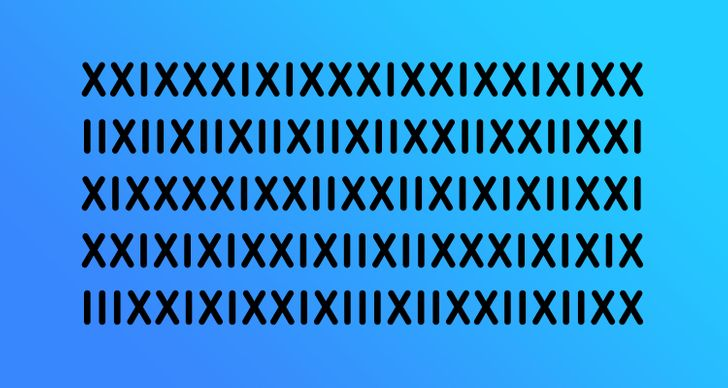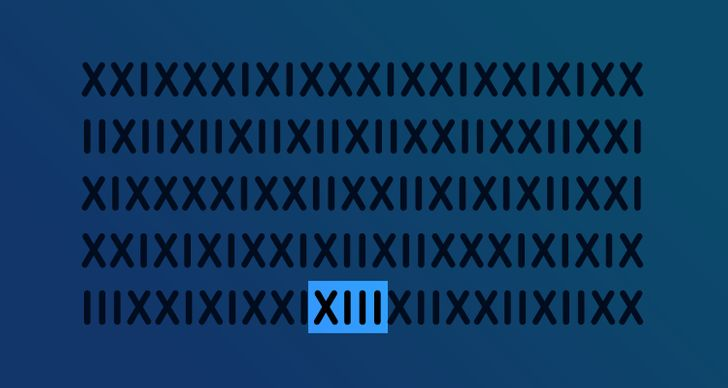### 14. Find the odd bunny.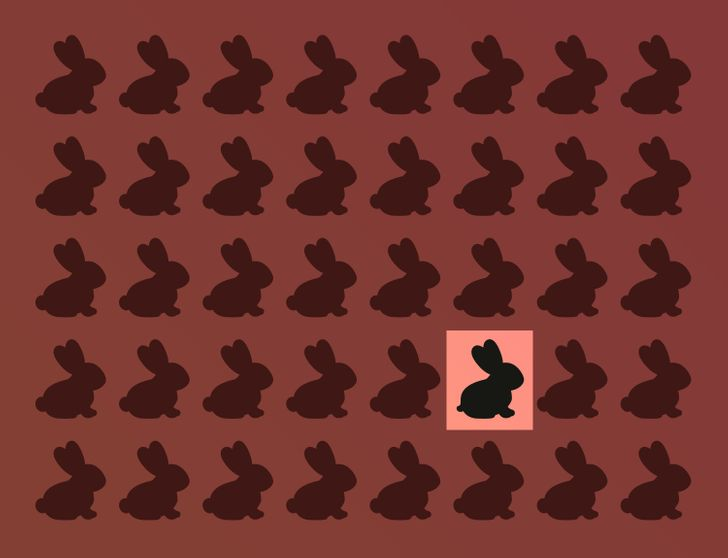### 15. Which one is the odd number?